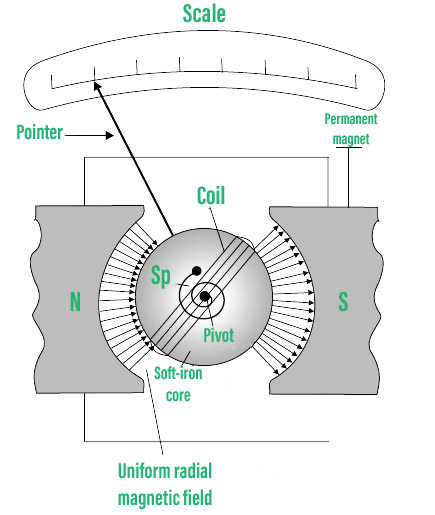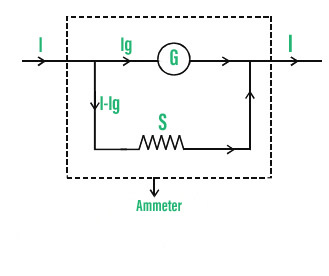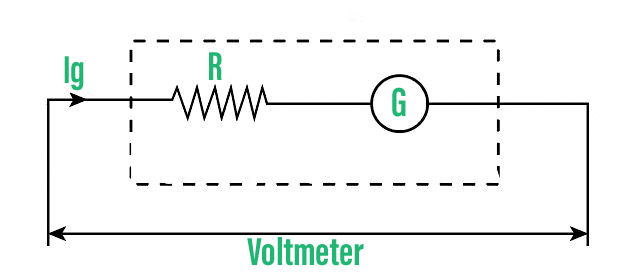GFG App
Open AppBrowser
Continue

Hans Christian Oersted discovered in 1820 that a current-carrying conducting wire produces a magnetic field around it. His findings from his experiments are as follows:

1. The magnetic compass needle is aligned tangent to an imaginary circle centered on the current-carrying cable.
2. When the current is reversed, the needle’s orientation is reversed as well.
3. The strength of the current flowing through the wire determines the needle’s deflection.

Magnetic fields mediate a class of physical properties known as magnetism. A magnetic field is created by electric currents and the magnetic moments of elementary particles, which operate on other currents and magnetic moments. Electromagnetism is a multifaceted phenomenon that includes magnetism. The most well-known effects occur in ferromagnetic materials, which are highly attracted to magnetic fields and can be magnetized to become permanent magnets that generate magnetic fields.

### What is Torque?

The force that can cause an object to twist along an axis is measured as torque. In linear kinematics, force is what causes an object to accelerate. Torque is also responsible for the angular acceleration. As a result, torque can be defined as the linear force’s rotational equivalent. The axis of rotation is the point at which the item rotates. Torque is the property of a force to turn or twist.

The torque might be static or dynamic. A torque that does not cause an angular acceleration is known as static torque.

e.g.:

• When a person pushes a closed door, the door remains static because it does not rotate despite the force applied.
• Because there is no acceleration, pedaling a bicycle at a steady speed is likewise an example of static torque.

Torque produced on a Rectangular Current loop in a Uniform Magnetic Field

When a rectangular loop with N turns of area A each carries a continuous current I and is put in a uniform magnetic field B so that the loop’s normal to the plane makes an angle with the magnetic field’s direction, the loop experiences a torque whose magnitude is given by:

τ= NIAB sin θ

The magnetic dipole moment of the current loop is defined as,

|m| = NIA

The magnetic dipole moment of a current-carrying loop can be calculated as the product of the loop’s current and total area, i.e.

M = I . (NA)

Due to the current in the loop, the magnetic dipole moment is a vector quantity with a direction parallel to the magnetic field direction.

### Moving Coil Galvanometer

A moving coil galvanometer is an instrument for measuring electric currents. It’s a sensitive electromagnetic gadget that can detect extremely low currents, down to a few microamperes.

There are two primary types of moving-coil galvanometers:

• A galvanometer with suspended coils
• A galvanometer with a pivoting coil or a Weston galvanometer

Principle

Magnetic torque is experienced when a current-carrying coil is put in an external magnetic field. The angle through which the coil is deflected due to the magnetic torque effect is proportional to the coil’s current magnitude.

Construction

The moving coil galvanometer is made consisting of a rectangular coil with several turns that are wound on a metallic frame and is usually constructed of thinly insulated or fine copper wire. The coil rotates freely around a fixed axis. The coil is suspended in a uniform radial magnetic field using a phosphor-bronze strip coupled to a moveable torsion head.

Conductivity and a low torsional constant are two important qualities of the material used to suspend the coil. To boost the strength of the magnetic field and make it radial, a cylindrical soft iron core is symmetrically positioned inside the coil. The coil’s lower half is connected to a phosphor-bronze spring with a modest number of turns. The spring’s other end is attached to binding screws.

The spring is employed to generate a counter torque that serves to balance the magnetic torque and produce a consistent angular deflection. The deflection of the coil is measured using a plane mirror attached to the suspension wire, as well as a lamp and scale arrangement. The scale’s zero points are in the middle.Pictorial representation of Galvanometer

Working

Allow me to pass through a rectangular coil with n turns and an area of A in cross-section. When the coil is placed in a uniform radial magnetic field B, it experiences a torque. Consider a rectangular coil with a length of l and a width of b with a single turn ABCD. The coil is suspended in a magnetic field of strength B, with the plane of the coil parallel to the field. The sides AB and DC are not subjected to any magnetic field’s effective force because they are parallel to the field’s direction.

The perpendicular to the field’s direction sides AD and BC are subjected to an effective force F, which is given by,

F = BI

We may deduce that the forces acting on AD and BC are in opposite directions using Fleming’s left-hand rule. When equal and opposite forces F, known as the pair, act on the coil, a torque is produced. The coil deflects as a result of the torque.

We know that,

Torque (τ) = force x perpendicular distance between the forces

or

τ = F × b

Using the value of F that we already know as a substitute,

Torque τ acting on single-loop ABCD of the coil = BI × b

where l × b is the area A of the coil,

Hence the torque acting on n turns of the coil is given by,

τ = n I A B

The coil rotates as a result of the magnetic torque, and the phosphor bronze strip twists. The spring S linked to the coil, in turn, provides a counter torque or restoring torque k, resulting in a constant angular deflection.

Under equilibrium condition:

kθ = nIAB

The torsional constant of the spring is denoted by the letter k (restoring couple per unit twist). The deflection or twist is measured by a pointer attached to the suspension wire that indicates a value on a scale.

θ = (nAB / k) I

Therefore,

θ ∝ I

For a given galvanometer, the quantity nAB / k is a constant. As a result, it is clear that the deflection of the galvanometer is proportional to the current flowing through it.

### Sensitivity Of Moving Coil Galvanometer

The ratio of change in deflection of the galvanometer to change in current in the coil is the broad definition of sensitivity experienced by a moving coil galvanometer.

S = dθ/ dI

If a galvanometer shows a larger deflection for a modest amount of current, it has a higher sensitivity. Current sensitivity and voltage sensitivity are the two types of sensitivity.

• Current sensitivity: The deflection (θ) per unit current (I) is known as current sensitivity θ/I

θ/I = nAB/k

• Voltage sensitivity: Voltage sensitivity θ/V is the amount of deflection (θ) per unit voltage(V)

θ/V= (nAB/Vk) I

The effective resistance in the circuit is denoted by the letter R. It’s worth remembering that voltage sensitivity is equal to current sensitivity/coil resistance. As a result, assuming R remains constant, voltage sensitivity ∝ current sensitivity.

The figure of Merit of a Galvanometer: It’s the proportion of the full-scale deflection current of the instrument to the number of graduations on the scale. It’s also the reciprocal of the current sensitivity of a galvanometer.

Factors affecting Sensitivity of a Galvanometer:

1. The number of turns in the coil N and the coil’s area A.
2. The strength of the magnetic field B.
3. k/nAB is the magnitude of the pair per unit twist.

### Applications of Galvanometer

Because the moving coil galvanometer is such a sensitive instrument, it may be used to detect the presence of current in any circuit. When a galvanometer is used in a Wheatstone’s bridge circuit, the pointer in the galvanometer exhibits no deflection, indicating that no current is flowing through the device. Depending on the direction of the current, the pointer deflects to the left or right.

By connecting it in parallel to low resistance, the galvanometer can be used to measure:

• The value of current in the circuit.
• The voltage can be increased by connecting it in series with a high resistance resistor.

### Conversion of Galvanometer to Ammeter

By connecting a galvanometer in parallel with a low resistance known as shunt resistance, a galvanometer can be transformed into an ammeter. Depending on the ammeter’s range, a suitable shunt resistance is selected.

Let’s now consider Rg as the Resistance of the galvanometer, G as Galvanometer coil, I represents the total current passing through the circuit, Ig represents the total current passing through the galvanometer which corresponds to full-scale reading, and Rs shows the value of shunt resistance.

The current via the shunt resistance, when current Ig passes through the galvanometer is given by,

Is = I – I

Due to the parallel nature of their connection, the voltages across the galvanometer and shunt resistance are equal.

Therefore,

Rg × Ig = (I –  Ig) × RsGalvanometer to Voltmeter

### Conversion of Galvanometer to Voltmeter

By putting a galvanometer in series with large resistance, it can be transformed into a voltmeter. Depending on the voltmeter’s range, a suitable high resistance is selected.

Let’s now consider Rg as the Resistance of the galvanometer, G as Galvanometer coil, I represents the total current passing through the circuit, Ig represents the total current passing through the galvanometer which corresponds to full-scale reading, and V shows the voltage drop across the series connection of galvanometer and high resistance.

The voltage drop across the branch ab is given by when current Ig travels through the series combination of the galvanometer and the high resistance R.

V = Rg × Ig + R × IgGalvanometer to voltmeter

Advantages of a Moving Coil Galvanometer are:

• Provides high Sensitivity.
• Stray magnetic fields have little effect on it.
• It has a high torque-to-weight ratio.
• High precision and dependability.

Disadvantages of a Moving Coil Galvanometer are:

• Only direct currents can be measured using it.
• Errors develop as a result of causes such as instrument aging, permanent magnets, and mechanical stress damage to the spring.

### Sample Problems

Problem 1: For a current of 4mA, a galvanometer coil with a resistance of 40 shows full range deflection. How can this galvanometer be transformed into a 0-12 V voltmeter?

Solution:

As we know that V = IG (RG + R)

R = V/ IG – RG

= (12/ (4×10-3)) – 40

R = 2960 Ω

Problem 2: What is the purpose of putting a soft iron cylindrical core inside the moving coil galvanometer?

Solution:

The inside of the galvanometer has a cylindrical soft iron core that boosts the magnetic field strength and so improves the instrument’s sensitivity. It also makes the magnetic field radial, ensuring that the angle between the coil’s plane and the magnetic lines of force is always zero during rotation.

Problem 3: With a resistance of 0.1 Ω, a moving coil galvanometer of resistance of 100 Ω is used as an ammeter. The galvanometer’s maximum deflection current is 100 μA. Find the current in the circuit that causes the ammeter to deflect the most.

Solution:

It is given that, Rg =100Ω , Rs= 0.1Ω, Ig =100μA

We know that, Rg x Ig= (I- Ig) x Rs

Therefore I = (Rg  x Ig+ Ig x Rs)/ Rs

I= (1+Rg/ Rs) x Ig

Substituting the given values, we get I= 100.1mA

Problem 4: What is the principle of Moving coil galvanometer?

Solution:

Magnetic torque is experienced when a current-carrying coil is put in an external magnetic field. The angle through which the coil is deflected due to the magnetic torque effect is proportional to the coil’s current magnitude.

Problem 5: Write Advantages of moving coil galvanometer

Solution:

• Sensitivity is high.
• Stray magnetic fields have little effect on it.
• It has a high torque-to-weight ratio.
• High precision and dependability.

My Personal Notes arrow_drop_up
Related Tutorials